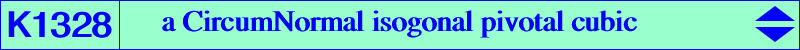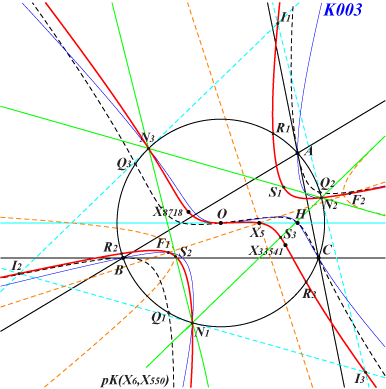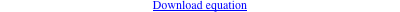too complicated to be written here. Click on the link to download a text file.X(3), X(5), X(8718), X(33541) vertices of the CircumNormal triangle infinite points of pK(X6, X550) further details below Geometric properties :K1328 is the isogonal pK with pivot X(5) with respect to the CircumNormal triangle. It is a member of the pencil of cubics which contains K003, K725, K1267. This pencil is stable under this isogonal conjugation and K725, K1328 are the only two self-isogonal cubics. K1267 is the transform of K003. Points on K1328 N1, N2, N3 : vertices of the CircumNormal triangle Q1, Q2, Q3 : points on (O) and pK(X6, X550), not on K1328 R1, R2, R3 : their antipodes on (O) S1, S2, S3 : vertices of the cevian triangle of X(5) in the CircumNormal triangle I1, I2, I3 : excenters of the CircumNormal triangle F1, F2 : real foci of the inconic of the CircumNormal triangle with center X(5)